## 领导把降温说成降价 帝豪“含泪”送清凉

2017年07月27日 11:14 来源：www.chextx.com 超过：次关注

最近吉利帝豪对外公布:凡在7月-9月购车，现金直减一万元，同时两年免息，最高可享受4000元补贴。消息一出，圈内炸裂，很多汽车圈内人直呼“连续六年销冠还需要这种操作”？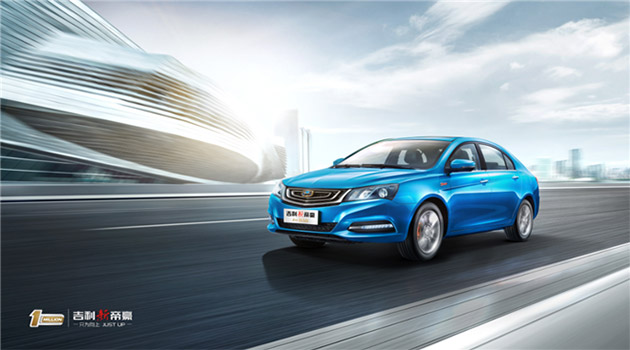不过这一波“清凉”来得也是一波三折，颇为曲折，情况据说是这样的——

最近帝豪销量火爆，领导召集各部门小弟开会分配下季度销售任务。有位分管市场的小弟在会上建议领导趁着帝豪上市八周年推出优惠政策，一来回馈消费者，二来缓解下自己销售压力。领导听完，只说了一句：吃饭！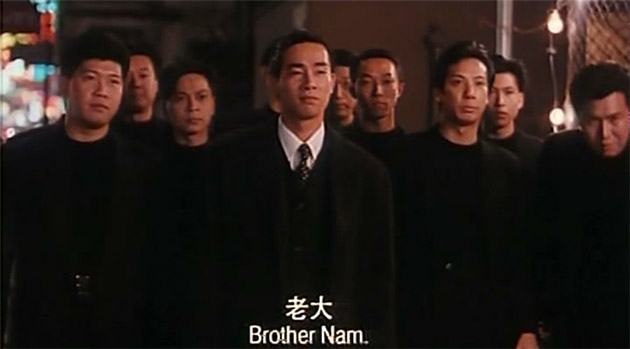领导一向十分低调，凡在公司上班必去食堂吃饭。这天杭州41度，去食堂的路上，领导一言不发，分管市场的小弟跟在后面汗流直下，隐约意识到自己的建议领导并不满意。

平常不到5分钟的路领导今天晃了20分钟还没到，快到一半的时候,只觉头晕目眩，噗通一声倒地。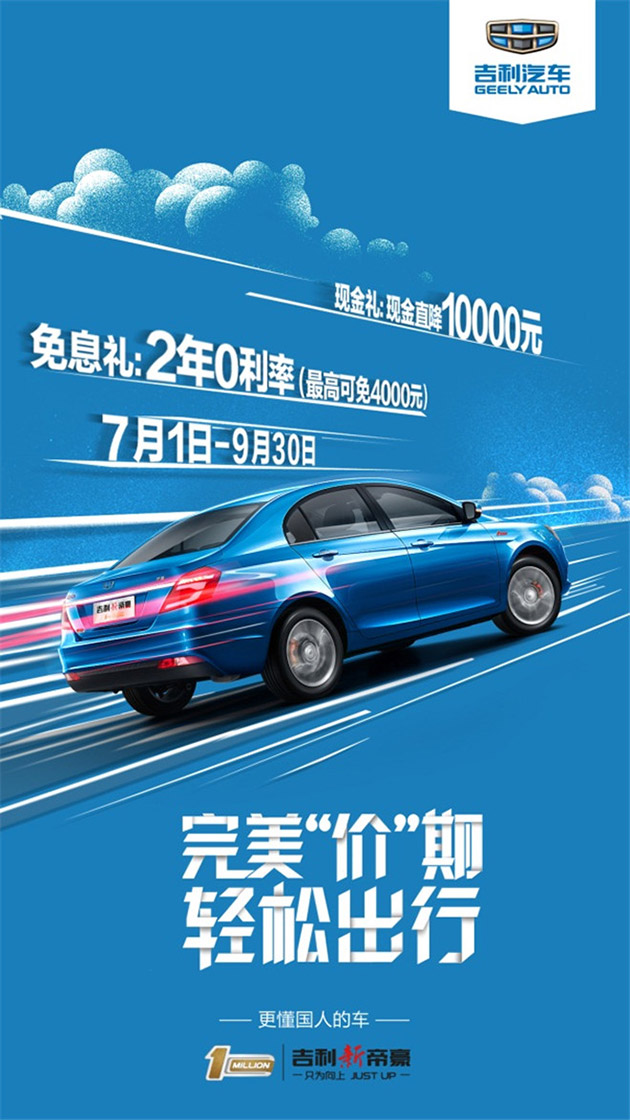分管市场的小弟眼疾手快，迅速上去抱住领导猛按人中，各位小弟一看不好，也迅速围上来，里三层外三层围的密不透风，领导挣扎着，断断续续地说到：“散开散开，快给我降，降….”话没说完晕了过去。

一阵慌乱之后，领导被120接走后。各位小弟一合计，刚才领导“降”子说了一半，肯定是领导一路上斟酌再三，最终同意了促销的建议，既然这样，马屁拍晚了没事，工作做迟了可就不好交代。于是各路广告、促销单铺天盖地，不到3个小时，一场轰轰烈烈的购车减1万，两年免息享4000的优惠活动迅速抢占头条。

分管市场的小弟因为领导采纳了自己的提议十分得意，第二天兴致冲冲赶到医院看望领导并汇报工作。“领导，昨天您安排降价优惠的工作我们已经全部部署下去，现在已经是网络头条。”

听说帝豪成功抢占头条，领导什么话也没说，默默点了根烟，招招手让大家都出去。最后一位小弟带上门的一刹，领导吐了口烟，只能含泪无语凝噎:都是“高烤”惹的祸啊……

----------------------------华丽丽的故事分割线-----------------------

小笑怡情，大笑养生；酷暑难忍，以上故事纯属逗乐，优惠大酬宾才真是清凉！

今年大暑以后，江浙沪包邮升级到“江浙沪包热”，动辄40+的温度，江浙沪人民终于可以硬气的问一声：还有谁？

冬冷夏热本是自然规律，但据气象预报，本周江浙沪地区极限温度将达到42度。正常人体温度超过39度既是高烧。现在，只要你敢出门分分钟高烧40度以上，运气好的话，打破个人记录也不是不可能。无怪网友直呼“我这条命是空调给的。”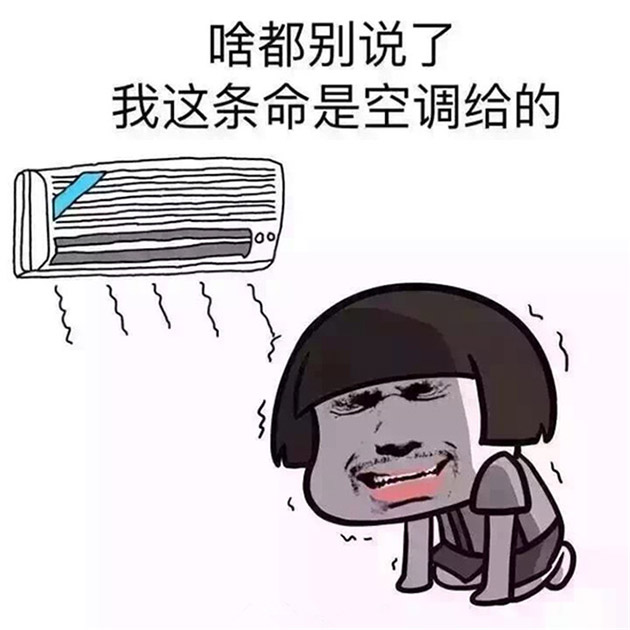可是出门怎么办？动辄接近50度的地面上，人人都一块行走的烤肉。更别提拥挤的公交或者地铁。假如一辆公交只装10个人，按照人均37度计算，整车的温度将达到可怕的370度……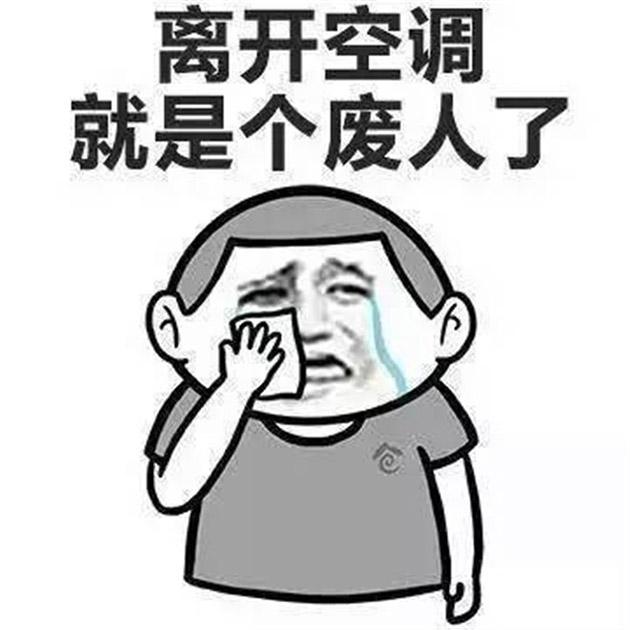吉利帝豪搭载的全自动恒温空调，就成为夏日出门的降温神器。它具备快速制冷能力并可自动调节车内温度，当车内温度保持设定的状态，制冷压缩机停止工作，避免不必要的油耗，清凉节油两不误。

7月-9月，凡是感觉离开空调就会成为废人的用户，购买吉利新帝豪，将可以享受一万四千元重新做人优惠补贴，记住，重新做人的机会不是每次都有哦

#### 相关文章

﻿
• 快速找车
• 选择品牌
• 选择品牌
• A  奥迪
• A  阿斯顿·马丁
• A  阿尔法·罗密欧
• B  宝沃
• B  布加迪
• B  巴博斯
• B  保时捷
• B  宾利
• B  奔驰
• B  宝马
• B  本田
• B  别克
• B  标致
• B  比亚迪
• B  宝骏
• B  北汽制造
• B  北汽新能源
• B  北汽幻速
• B  北汽威旺
• B  北京汽车
• B  奔腾
• B  北汽绅宝
• B  北汽昌河
• C  长安欧尚
• C  长安
• C  长安凯程
• C  长城
• D  大众
• D  道奇
• D  DS
• D  东南
• D  东风风神
• D  东风风行
• D  东风小康
• D  东风风度
• D  东风
• F  福特
• F  丰田
• F  菲亚特
• F  法拉利
• F  福田
• F  福迪
• F  福汽启腾
• G  观致
• G  广汽传祺
• G  广汽吉奥
• G  GMC
• H  红旗
• H  汉腾汽车
• H  哈弗
• H  哈飞
• H  海格
• H  海马
• H  华颂
• H  黄海
• H  华泰
• H  恒天
• J  捷途
• J  几何汽车
• J  捷达
• J  吉利汽车
• J  捷豹
• J  Jeep
• J  江淮
• J  江铃
• J  金杯
• J  九龙
• J  金旅
• K  凯翼
• K  凯迪拉克
• K  克莱斯勒
• K  科尼塞克
• K  卡威
• K  开瑞
• L  路虎
• L  林肯
• L  劳斯莱斯
• L  兰博基尼
• L  雷克萨斯
• L  铃木
• L  领克
• L  雷诺
• L  理念
• L  力帆
• L  莲花汽车
• L  猎豹
• L  路特斯
• L  陆风
• M  马自达
• M  MG
• M  MINI
• M  玛莎拉蒂
• M  摩根
• M  迈凯轮
• N  纳智捷
• O  欧拉
• O  欧宝
• O  讴歌
• O  欧朗
• Q  奇瑞
• Q  起亚
• Q  启辰
• R  日产
• R  荣威
• R  瑞麒
• S  SERES赛力斯
• S  三菱
• S  斯威汽车
• S  萨博
• S  smart
• S  斯柯达
• S  斯巴鲁
• S  思铭
• S  双龙
• S  上汽大通
• S  双环
• T  特斯拉
• T  腾势
• W  蔚来
• W  沃尔沃
• W  WEY
• W  五菱汽车
• W  五十铃
• W  威兹曼
• W  威麟
• X  现代
• X  雪佛兰
• X  星途
• X  雪铁龙
• X  小鹏汽车
• X  西雅特
• Y  一汽
• Y  英菲尼迪
• Y  英致
• Y  依维柯
• Y  野马汽车
• Y  永源
• Z  众泰
• Z  中华
• Z  中兴
• Z  知豆
• 选择车系
• 选择车系
• 车型对比
• 选择品牌
• 选择品牌
• A  奥迪
• A  阿斯顿·马丁
• A  阿尔法·罗密欧
• B  宝沃
• B  布加迪
• B  巴博斯
• B  保时捷
• B  宾利
• B  奔驰
• B  宝马
• B  本田
• B  别克
• B  标致
• B  比亚迪
• B  宝骏
• B  北汽制造
• B  北汽新能源
• B  北汽幻速
• B  北汽威旺
• B  北京汽车
• B  奔腾
• B  北汽绅宝
• B  北汽昌河
• C  长安欧尚
• C  长安
• C  长安凯程
• C  长城
• D  大众
• D  道奇
• D  DS
• D  东南
• D  东风风神
• D  东风风行
• D  东风小康
• D  东风风度
• D  东风
• F  福特
• F  丰田
• F  菲亚特
• F  法拉利
• F  福田
• F  福迪
• F  福汽启腾
• G  观致
• G  广汽传祺
• G  广汽吉奥
• G  GMC
• H  红旗
• H  汉腾汽车
• H  哈弗
• H  哈飞
• H  海格
• H  海马
• H  华颂
• H  黄海
• H  华泰
• H  恒天
• J  捷途
• J  几何汽车
• J  捷达
• J  吉利汽车
• J  捷豹
• J  Jeep
• J  江淮
• J  江铃
• J  金杯
• J  九龙
• J  金旅
• K  凯翼
• K  凯迪拉克
• K  克莱斯勒
• K  科尼塞克
• K  卡威
• K  开瑞
• L  路虎
• L  林肯
• L  劳斯莱斯
• L  兰博基尼
• L  雷克萨斯
• L  铃木
• L  领克
• L  雷诺
• L  理念
• L  力帆
• L  莲花汽车
• L  猎豹
• L  路特斯
• L  陆风
• M  马自达
• M  MG
• M  MINI
• M  玛莎拉蒂
• M  摩根
• M  迈凯轮
• N  纳智捷
• O  欧拉
• O  欧宝
• O  讴歌
• O  欧朗
• Q  奇瑞
• Q  起亚
• Q  启辰
• R  日产
• R  荣威
• R  瑞麒
• S  SERES赛力斯
• S  三菱
• S  斯威汽车
• S  萨博
• S  smart
• S  斯柯达
• S  斯巴鲁
• S  思铭
• S  双龙
• S  上汽大通
• S  双环
• T  特斯拉
• T  腾势
• W  蔚来
• W  沃尔沃
• W  WEY
• W  五菱汽车
• W  五十铃
• W  威兹曼
• W  威麟
• X  现代
• X  雪佛兰
• X  星途
• X  雪铁龙
• X  小鹏汽车
• X  西雅特
• Y  一汽
• Y  英菲尼迪
• Y  英致
• Y  依维柯
• Y  野马汽车
• Y  永源
• Z  众泰
• Z  中华
• Z  中兴
• Z  知豆
• 选择车系
• 选择车系
• 选择车型
• 选择车型
• 意见反馈